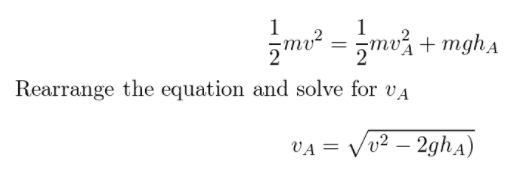# Mechanical energy- how to solve for v

Woopa
Homework Statement:
Mechanical energy. Solve for v
Relevant Equations:
1/2mv^2 + mgh
Hi there,

I am doing a mechanical energy question. I think the solution is simple but I'm stuck on an algebra step.This is the solution but I am really not sure how they have simplified down to Va.

For example I tried to factor out the m resulting in

1/2mv^2=m(1/2va^2+gh)

Then I cancel the m

1/2v^2= 1/2va^2+gh

From here I am not really sure what they did to arrive at the final answer

Homework Helper
Gold Member
2022 Award
Homework Statement:: Mechanical energy. Solve for v
Relevant Equations:: 1/2mv^2 + mgh

Hi there,

I am doing a mechanical energy question. I think the solution is simple but I'm stuck on an algebra step.

View attachment 279825
This is the solution but I am really not sure how they have simplified down to Va.

For example I tried to factor out the m resulting in

1/2mv^2=m(1/2va^2+gh)

Then I cancel the m

1/2v^2= 1/2va^2+gh

From here I am not really sure what they did to arrive at the final answer
You can see they got rid of the factors of 1/2. How would you do that?
They also got vA on one side of the equation and everything else on the other , so do that.

Woopa
You can see they got rid of the factors of 1/2. How would you do that?
They also got vA on one side of the equation and everything else on the other , so do that.
I'm really not sure how they got rid of the factors of 1/2. Can you help me?

Getting VA to one side is easy enough for me. Starting with ½v^2=½VA^2+gh after factoring out m, I would then just subtract gh resulting in 1/2 v^2-gh= 1/2 VA^2.

Still really not sure how they got rid of the halfs. My first thought is to multiply by 2 but that doesn't give me the answer

Homework Helper
Gold Member
2022 Award
My first thought is to multiply by 2
It gets rid of the halves, so do it.
With vA alone on one side, what is your equation now?

Woopa
It gets rid of the halves, so do it.
With vA alone on one side, what is your equation now?

If I multiply by 2 and then sqrt I end up with

VA=√ v^2-gh

I'm just missing the 2gh

Homework Helper
Gold Member
2022 Award
If I multiply by 2 and then sqrt I end up with

VA=√ v^2-gh

I'm just missing the 2gh
Then you did not get rid of the halves correctly. Retry that step.

Woopa
Then you did not get rid of the halves correctly. Retry that step.
It's just clicked! Thank you for the prompts I've got it